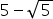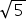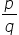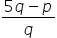Maths-
General
Easy

Question

# Prove thatis an irrational number.

## The correct answer is: YES an irrational number.

### Hint:The real numbers which cannot be expressed in the form of p/q(where p and q are integers and q ≠ 0) are known as irrational numbers. In the given question we are asked to prove if 5-is an irrational number. To do so we use the method of contradiction.SolutionLet's assume that 5-is a rational number.Step 1 of 2:If 5-is rational, that means it can be written in the form of p/q, where p and q integers q ≠ 0.SO 5 -=5 -==Step 2 of 2:Here, we see thatis a rational number. Butis an irrational number. So our assumption is wrong.Final Answer: Asis an irrational number. Somust also be an irrational number. Thus, our assumption is wrong. Hence, 5-is an irrational number#### With Turito Foundation.#### Get an Expert Advice From Turito.